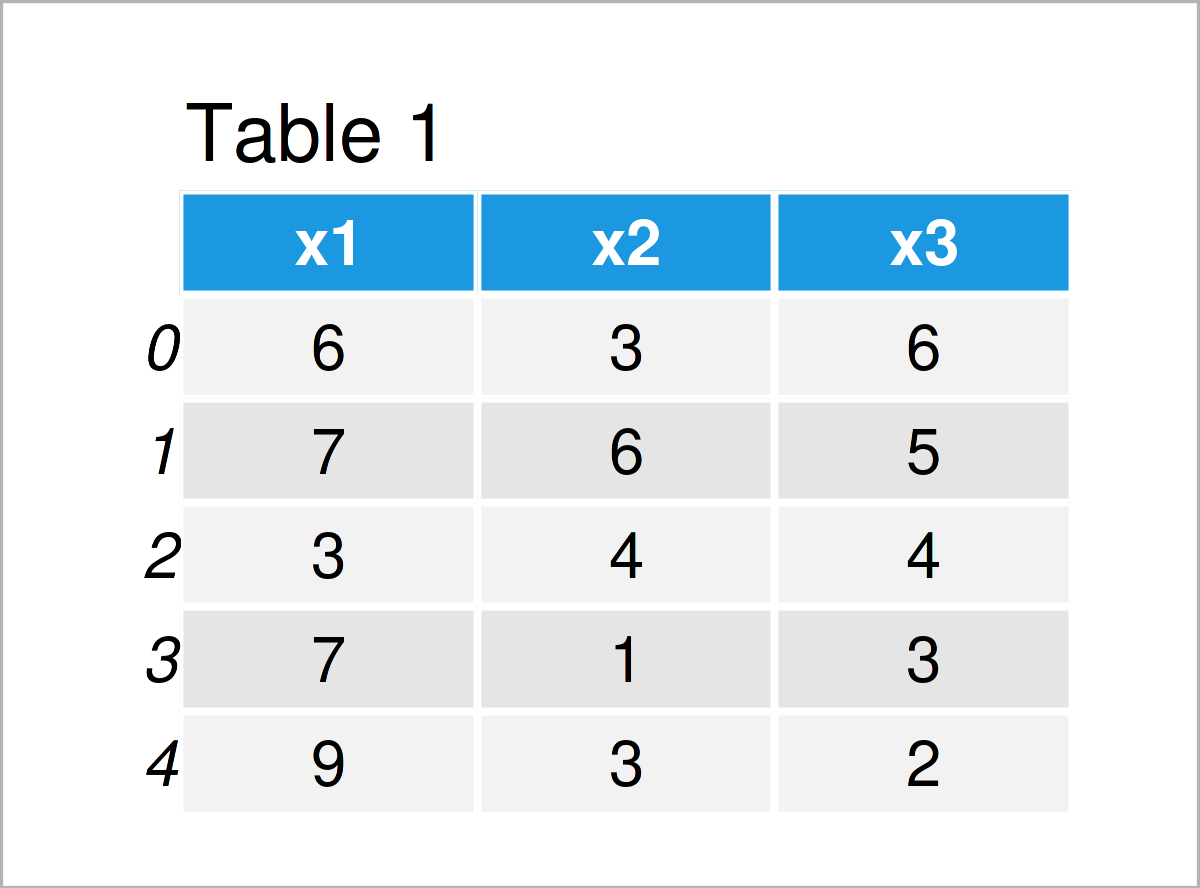# Check if pandas DataFrame is Empty in Python (2 Examples)

In this Python tutorial you’ll learn how to test whether a pandas DataFrame is empty.

The post consists of this content:

Let’s dive into it!

## Example 1: Test whether pandas DataFrame is Empty (Non-Empty Data)

The Python programming syntax below shows how to how to evaluate whether a pandas DataFrame contains any values.

First, we need to load the pandas library:

`import pandas as pd                             # Load pandas library`

Next, we have to create a pandas DataFrame in Python:

```data1 = pd.DataFrame({'x1':[6, 7, 3, 7, 9],    # Create example DataFrame
'x2':[3, 6, 4, 1, 3],
'x3':range(6, 1, - 1)})
print(data1)                                   # Print example DataFrame```The output of the previous syntax is shown in Table 1 – We have created a pandas DataFrame containing three columns and five non-empty rows.

We can now use the empty attribute of our pandas DataFrame to return a logical indicator that shows whether our data is empty:

```print(data1.empty)                             # Test if DataFrame is empty
# False```

The previous Python code has returned the logical indicator False, i.e. our data set is not empty.

## Example 2: Test whether pandas DataFrame is Empty (Empty Data)

In Example 1 we have used a non-empty data matrix as basement. For comparison, let’s create an empty DataFrame in Python:

```data2 = pd.DataFrame()                         # Create empty DataFrame
print(data2)                                   # Print empty DataFrame
# Empty DataFrame
# Columns: []
# Index: []```

Now, we can use the empty attribute once again:

```print(data2.empty)                             # Test if DataFrame is empty
# True```

This time the logical indicator True is returned, i.e. our data set is empty.

## Video & Further Resources on this Topic

Do you need more explanations on how to test whether a pandas DataFrame is empty? Then you might have a look at the following video of the Statistics Globe YouTube channel.

Please accept YouTube cookies to play this video. By accepting you will be accessing content from YouTube, a service provided by an external third party.If you accept this notice, your choice will be saved and the page will refresh.

If you need more explanations on pandas DataFrames, I can recommend watching the following video on the YouTube channel of Corey Schafer. He shows different tips and tricks on how to handle pandas DataFrames in the video.

Please accept YouTube cookies to play this video. By accepting you will be accessing content from YouTube, a service provided by an external third party.If you accept this notice, your choice will be saved and the page will refresh.

Furthermore, you might want to have a look at the related Python programming articles on this homepage. Please find a selection of tutorials below.

On this page you have learned how to check whether a pandas DataFrame is empty in the Python programming language. Let me know in the comments section, in case you have further questions.

Subscribe to the Statistics Globe Newsletter